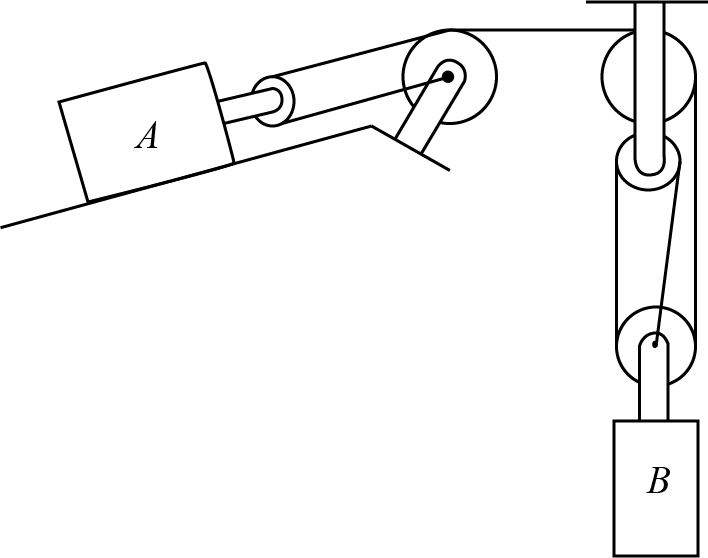# #In the moment shown above, $$B$$ is travelling downwards at $$v = {{params.v_b}}m/s$$, but accelerating upwards at $$a = {{params.a_b}}m/s^2$$.

## Part 1#

Determine the velocity of block $$A$$. Positive if downhill, negative if uphill.

Please enter an integer value in $${{ params.vars.units_v }}$$.

## Part 2#

Determine the acceleration of block $$A$$. Positive if downhill, negative if uphill.

Please enter an integer value in $${{ params.vars.units_a }}$$.